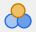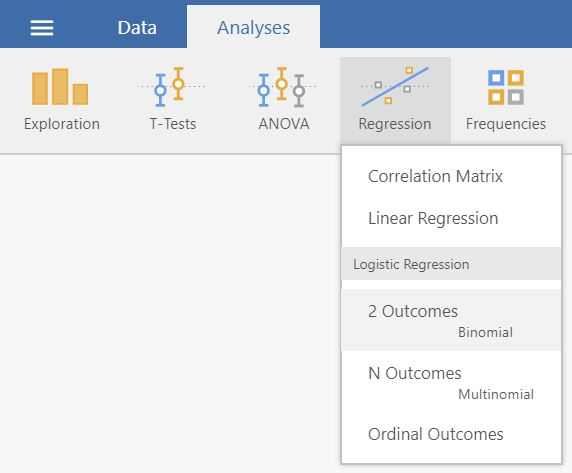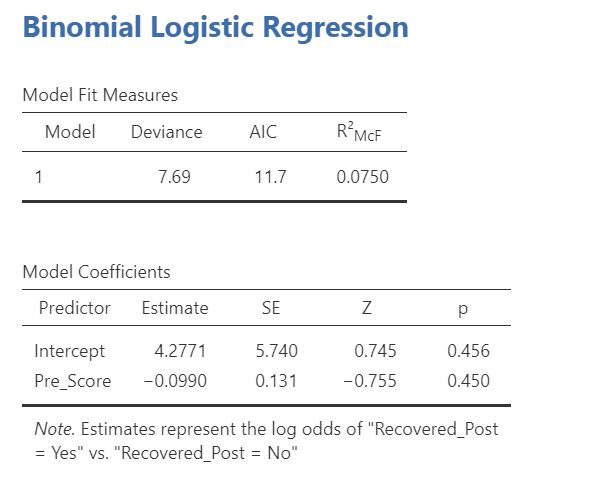# 12 Logistic Regression

#### How to perform a logistic regression in jamovi:

1. You need one continuous predictor variable and one categorical (nominal or ordinal) outcome variable. Make sure that the measurement levels are set10 so that the continuous variable is marked withand the grouping variable is marked with.
A correct setup should look similar to this:2. Logistic regression can be found by selecting `Analyses` -> `Regression`. If the outcome variable is nominal (as in the above image), select `2 Outcomes` if it has 2 outcomes, or `N outcomes`if it has more than 2 outcomes. If the outcome variable is ordinal (e.g. low, medium, high), select `Ordinal Outcomes`.3. Drag and drop your outcome variable to Dependent Variable and your predictor to Covariates.4. The result is shown in the right panel:1. This is demonstrated in section 2.